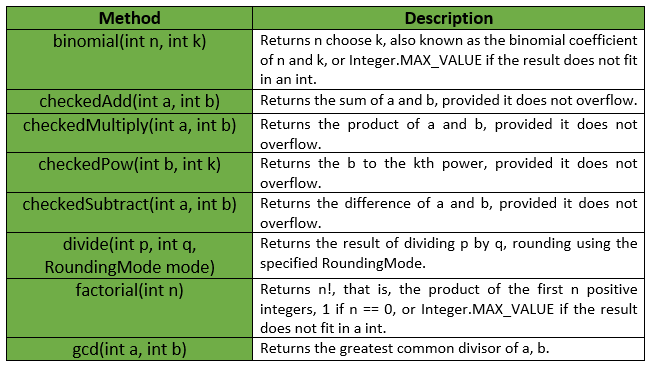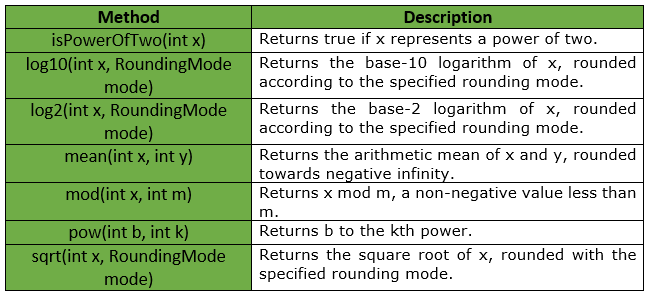Open in App
Not now

# IntMath Class | Guava | Java

• Last Updated : 25 May, 2018

Introduction : IntMath is used to perform mathematical operations on Integer values. Basic standalone math functions are divided into the classes IntMath, LongMath, DoubleMath, and BigIntegerMath based on the primary numeric type involved. These classes have parallel structure, but each supports only the relevant subset of functions.

Declaration : The declaration for com.google.common.math.IntMath class is :

```@GwtCompatible(emulated = true)
public final class IntMath
extends Object
```

Below table shows some of the methods provided by IntMath Class of Guava :Exceptions :

• log2 : IllegalArgumentException if x <= 0
• log10 : IllegalArgumentException if x <= 0
• pow : IllegalArgumentException if k < 0
• sqrt : IllegalArgumentException if x < 0
• divide : ArithmeticException if q == 0, or if mode == UNNECESSARY and a is not an integer multiple of b
• mod : ArithmeticException if m <= 0
• gcd : IllegalArgumentException if a < 0 or b < 0
• checkedAdd : ArithmeticException if a + b overflows in signed int arithmetic
• checkedSubtract : ArithmeticException if a – b overflows in signed int arithmetic
• checkedMultiply : ArithmeticException if a * b overflows in signed int arithmetic
• checkedPow : ArithmeticException if b to the kth power overflows in signed int arithmetic
• factorial : IllegalArgumentException if n < 0
• binomial : IllegalArgumentException if n < 0, k n

Some other methods provided by IntMath Class of Guava are :Example 1 :

 `// Java code to show implementation of``// IntMath Class of Guava``import` `java.math.RoundingMode;``import` `com.google.common.math.IntMath;`` ` `class` `GFG {`` ` `    ``// Driver code``    ``public` `static` `void` `main(String args[])``    ``{`` ` `        ``// Creating an object of GFG class``        ``GFG obj = ``new` `GFG();`` ` `        ``// Function calling``        ``obj.examples();``    ``}`` ` `    ``private` `void` `examples()``    ``{`` ` `        ``try` `{`` ` `            ``// exception will be thrown as 80 is not``            ``// completely divisible by 3``            ``// thus rounding is required, and``            ``// RoundingMode is set as UNNESSARY``            ``System.out.println(IntMath.divide(``80``, ``3``,``                           ``RoundingMode.UNNECESSARY));``        ``}``        ``catch` `(ArithmeticException ex) {``            ``System.out.println(``"Error Message is : "` `+ ``                                     ``ex.getMessage());``        ``}``    ``}``}`

Output :

```Error Message is : mode was UNNECESSARY, but rounding was necessary
```

Example 2 :

 `// Java code to show implementation of``// IntMath Class of Guava``import` `java.math.RoundingMode;``import` `com.google.common.math.IntMath;`` ` `class` `GFG {`` ` `    ``// Driver code``    ``public` `static` `void` `main(String args[])``    ``{``        ``// Creating an object of GFG class``        ``GFG obj = ``new` `GFG();`` ` `        ``// Function calling``        ``obj.examples();``    ``}`` ` `    ``private` `void` `examples()``    ``{`` ` `        ``// As 120 is divisible by 4, so``        ``// no exception is thrown``        ``System.out.println(IntMath.divide(``120``, ``4``,``                        ``RoundingMode.UNNECESSARY));`` ` `        ``// To compute GCD of two integers``        ``System.out.println(``"GCD is : "` `+ ``                             ``IntMath.gcd(``70``, ``14``));`` ` `        ``// To compute log to base 10``        ``System.out.println(``"Log10 is : "` `+``          ``IntMath.log10(``1000``, RoundingMode.HALF_EVEN));`` ` `        ``// To compute remainder``        ``System.out.println(``"modulus is : "` `+``                          ``IntMath.mod(``125``, ``5``));`` ` `        ``// To compute factorial``        ``System.out.println(``"factorial is : "` `+``                           ``IntMath.factorial(``7``));`` ` `        ``// To compute log to base 2``        ``System.out.println(``"Log2 is : "` `+ ``               ``IntMath.log2(``8``, RoundingMode.HALF_EVEN));`` ` `        ``// To compute square root``        ``System.out.println(``"sqrt is : "` `+``                    ``IntMath.sqrt(IntMath.pow(``12``, ``2``),``                          ``RoundingMode.HALF_EVEN));``    ``}``}`

Output :

```30
GCD is : 14
Log10 is : 3
modulus is : 0
factorial is : 5040
Log2 is : 3
sqrt is : 12
```